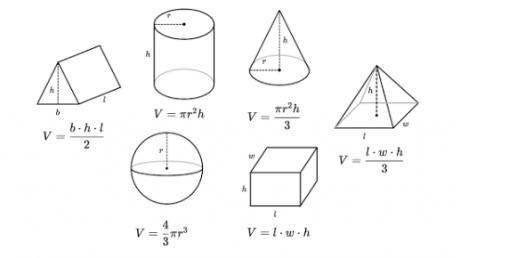# Heron’s Formula: Geometry Quiz! Test

15 Questions | Attempts: 7175
ShareSettingsHeron’s formula comes from geometry, and it gives the area of a triangle when the length of all three sides is known. It is not necessary to calculate angles of other distances in the triangle first. Heron’s formula was named after Hero of Alexandria. You must first calculate half of the triangle’s perimeter, and then you calculate the area. Give this quiz a try, and make sure you collect your certificate when you are done.Back to top